# Change Nickname

Current Nickname:

Certifications

Certificate Issued at Valid until Serial No. File
Serial No. Valid until

Not having one? Apply now! >>>

Invoice

ProductName CreateTime ID Price File
ProductName CreateTime ID Price File

# GraphSAGE

## Overview

GraphSAGE (SAmple and aggreGatE) is a general inductive framework. Instead of training individual embeddings for each node, it learns a function that generates embeddings by sampling and aggregating features from a node’s local neighborhood, thus can efficiently generate node embeddings for previously unseen data. GraphSAGE was proposed by W.H Hamilton et al. of Stanford University in 2017.

Related material of the algorithm:

## Basic Concept

### Transductive Framework and Inductive Framework

Most traditional graph embedding approaches (such as those algorithms based on matrix factorization or random walk) learn node embeddings by using the information of all nodes during the iterations; whenever there are unseen nodes join the network, the model has to be re-trained by using the whole dataset, as these transductive frameworks do not naturally generalize.

GraphSAGE, as a general inductive framework, it trains a set of aggregator functions instead individual embeddings for each node. Embeddings of newly joined nodes can be obtained according to the feature and structural information of nodes without re-iterate the whole training process. This inductive capability is essential for high-throughput, production machine learning systems.

### Embedding Generation Algorithm of GraphSAGEVisual illustration of the GraphSAGE sample (1) and aggregate (2) approach, the generated embeddings can be used in the downstream tasks such as label predication (3)

Assume that we have learned the parameters of K aggregator functions (AGGREGATEk), which aggregate information from node neighbors, as well as K weight matrices Wk, which are used to propagate information between different layers of the model or search depths. We train these parameters as described in GraphSAGE Train. The following is the GraphSAGE embedding generation process (i.e., forward propagation) in the minibatch setting:

#### 1. Sample neighborhood

• Fix the number of nodes that are sampled at each layer of neighborhood of the target nodes to Si (i = 1,2,...,K)
• For each target node: Perform uniform sampling at each layer of neighborhood of the node according to the set number, i.e., all neighbors have the same probability to be selected
• In case the number of neighbors at a certain layer is less than the set number, repeated sampling is performed until the set number of nodes are sampled

Fixed-size sampling is beneficial to adapt the algorithm for minibatch, as the memory and expected runtime of a single batch is fixed. The authors of GraphSAGE found that the K does not need to be big, practically it could achieve high performance with K = 2 and S1·S2 < 500.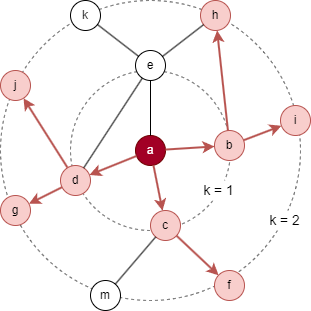Take the graph above as example, when the parameter `sample_size` is set as [5,3], sampling of the target node a is done from a to the farther neighborhood. Neighborhood set N2(a) = {b,c,d} is gained during the first round of sampling, and N1(a) = {f,g,h,i,j} during the second round.

The parameter `sample_size` is an array of integers, the length of the array is the maximum search depth K, elements in the array are the number of nodes sampled at layer-K, layer-K-1, ..., layer-1 successively.

Sampling is performed from layer-1 to layer-K, while the subscript order of the neighborhood set is exactly the opposite (K, K-1, ..., 1), which is to match the next step of aggregating feature information of neighbors from the outside in.

#### 2. Aggregate feature information from neighbors

For graph G = (V, E) and features for all nodes Xv (v∈V) are provided as input, Xv is formed by several properties of node and used as each node's initial representation vector: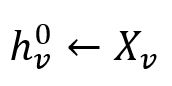Each iteration (k = K, ..., 2, 1) proceeds as follows:

• For all target nodes and nodes in the neighborhood sets (neighborhood sets with subscripts ≥ k are eliminated) of them, each node is denoted as u and calculated as below:

• Aggregate the (k-1)-th representations of the nodes in Nk(u) into a single neighborhood vector: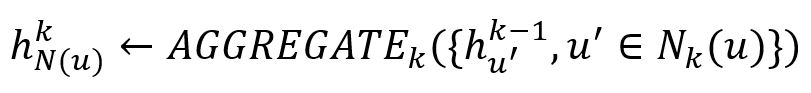• Concatenates the (k-1)-th representation of node u with the aggregated neighborhood vector, and this concatenated vector is fed through a fully connected layer with nonlinear activation function `σ` (such as Sigmoid function):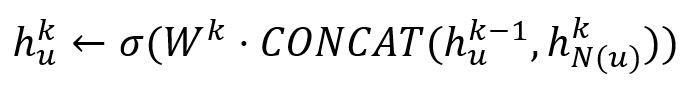• Normalize the representation of node u that is generated: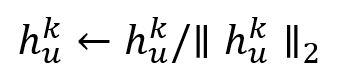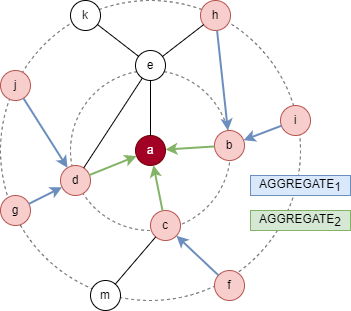For the target node a in the above example:

• Calculation of the first iteration: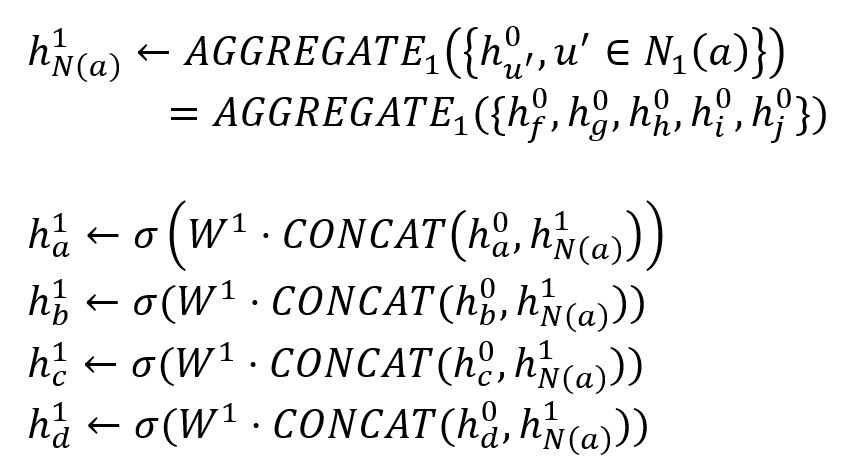• Calculation of the second iteration: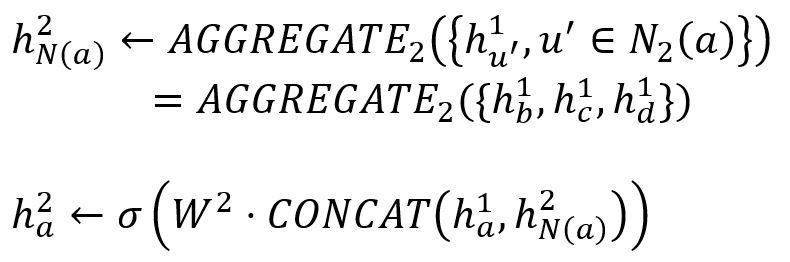## Special Case

### Lonely Node, Disconnected Graph

Lonely node has no adjacent node, hence it cannot generate feature information of any other nodes.

Nodes only generate feature information of neighbors in the same connected component.

### Self-loop Edge

Self-loop edges of node are ignored in the calculation.

### Directed Edge

For directed edges, GraphSAGE algorithm ignores the direction of edges but calculates them as undirected edges.

## Command and Configuration

• Command：`algo(graph_sage)`
• Configurations for the parameter `params()`:
Name Type
Default
Specification
Description
model_name string / / Name of the model trained by the algorithm `algo(graph_sage_train)`
model_task_id int / / Task ID of the algorithm that trained the model
ids []`_id` / / IDs of nodes to generate embeddings; all nodes to be selected if not set
node_property_names []`@<schema>?.<property>` Read from the model Node property, LTE needed Node property/properties to be used as features
edge_property_name `@<schema>?.<property>` Read from the model Numeric edge property, LTE needed Edge weight property/properties, schema can be either carried or not. During random walk, nodes only walk along edges with the specified properties and the probability of passing through these edges is proportional to the edge weight; if edge has multiple specified properties, the edge weight is the sum of these property values; the weight of all edges is 1 if not set
sample_size []int [25, 10] Read from the model Length of the array is the maximum search depth K, elements in the array are the number of nodes sampled at layer-K, layer-K-1, ..., layer-1 successively

## Algorithm Execution

#### 1. File Writeback

Not supported by this algorithm.

#### 2. Property Writeback

Configuration Writeback Content Type Data Type
property Embedding result Node property `string`

Example: Calculate embeddings of all nodes by GraphSAGE model named model_1, write the embedding results back to node property named embedding

``````algo(graph_sage).params({
model_name:'model_1'
}).write({
db:{
property_name:"embedding"
}
})
``````

#### 3. Statistics Writeback

This algorithm has no statistics.

### Direct Return

Not supported by this algorithm.

### Streaming Return

Not supported by this algorithm.

### Real-time Statistics

This algorithm has no statistics.